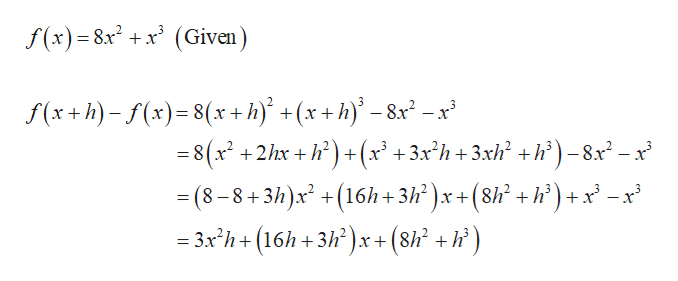# Find f(x+h)−f(x) given the following function.     f(x)=8x^2+x^3

Question
3 views

Find f(x+h)−f(x) given the following function.     f(x)=8x^2+x^3

check_circle

Step 1

Compute the subtraction of the function f(x+h...help_outlineImage Transcriptionclosef(x) 8x +x(Given f(x+h)-x)8(x+ h +(x+h-8x -x = 8(x2 +2/hxh +(x? +3x'h +3xh +h)-8x- x - (8-8+3/)x+(16h+3° )x + (8h° + }*°) + x° -x = 3x2h (16h 3h)x+ (8h2 +h) -3h)x +(8 fullscreen

### Want to see the full answer?

See Solution

#### Want to see this answer and more?

Solutions are written by subject experts who are available 24/7. Questions are typically answered within 1 hour.*

See Solution
*Response times may vary by subject and question.
Tagged in

### Other